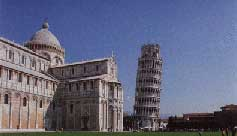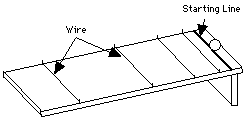# (13) The Way Things FallIndex12b. Orbital Motion12c. Venus transit (1)12d. Venus transit (2)12e. Venus transit (3)

Newtonian Mechanics13. Free Fall14. Vectors15. Energy15a. Atmospheric Energy
and Climate16. Newton's Laws17. Mass17a. Measuring Mass
in Orbit17b. Inertial balance18. Newton's 2nd Law18a. The Third Law18b. Momentum18c. WorkThe leaning tower of Pisa

Galileo was probably the first to look closely at the way objects fell down to Earth. Legend has it that he climbed to the top of the leaning tower of Pisa and from there dropped simultaneously heavy and light balls, noting that they hit the ground at the same time. He thus demonstrated, contrary to some ancient claims, that heavy and light objects ("bodies") fell at the same rate. Galileo's studies created great interest, because they applied not only to simple falls--such as the fall of an apple from a tree, said to have inspired Newton--but were also relevant to the very practical subject of the trajectory of cannonballs.

## Acceleration

A dropped object starts its fall quite slowly, but then steadily increases its velocity--accelerates--as time goes on. Galileo showed that (ignoring air resistance) heavy and light objects accelerated at the same constant rate as they fell, that is, their speed (or "velocity") increased at a constant rate. The velocity of a ball dropped from a high place increases each second by a constant amount, usually denoted by the small letter g (for gravity). In modern units (using the convention of algebra, that symbols or numbers standing next to each other are understood to be multiplied) its velocity is

at the start   -- 0 (zero)
after 1 second-- g meters/second
after 2 seconds-- 2g meters/second
after 3 seconds-- 3g meters/second

and so on. This is modified by the resistance of the air, which becomes important at higher speeds and usually sets an upper limit ("terminal velocity") to the fall velocity--a much smaller limit for someone using a parachute than one falling without.

The number g is close to 10--more precisely, 9.79 at the equator, 9.83 at the pole, and intermediate values in between--and is known as "the acceleration due to gravity." If the velocity increases by 9.81 m/s each second (a good average value), g is said to equal "9.81 meters per second per second" or in short 9.81 m/s2.

Suppose the ball was given an initial velocity u upwards or downwards. If distances down are chosen as positive, the velocity due to gravity will always be positive, while u will be positive if directed downwards, negative if upwards.

With this provision, observations show that u must be added everywhere to the velocity due to gravity, making the velocity in consecutive seconds (as in the list above)

u, u+g, u+2g, u+3g . . .

and in general, after t seconds, with gt meaning "g times t"

u + gt.

## The Distance Covered

The distance covered by the ball can be shown to be the same as the one covered if the ball has a constant "mean" velocity v(mean), equal to half the sum of the velocities at the start and at the finish. For the falling ball in the preceding example, after t seconds that would be

v(mean) = (1/2)[u + (u+gt)]

making the distance covered

distance = t v(mean) = ut + (1/2) gt2

## Galileo's Experiment

The book "The God Particle" by Nobel prize winner Leon Lederman with Dick Teresi (a good book, if you tolerate its irreverent attitude and corny humor) tells how Galileo showed that the downward motion of a ball due to gravity had a constant acceleration, and that starting from rest (u = 0) it covered distances which increased (as shown in the formula above) in proportion to the square of the time that has passed.Since a vertical fall was too fast for Galileo to measure accurately, he slowed it down by making the ball roll down an inclined board. Across the board, along to its surface, he strung a number of taut horizontal wires, making the ball sound a click whenever it jumped over one of them. Galileo then moved the wires up and down the board, until the clicks sounded evenly spaced.

If the acceleration now was a (much less than g) and the time t was measured in clicks, by the formula above, starting with the ball at rest (u= 0) one gets for the distance S covered

After one click S = (a/2) 12= a/ 2
After two     S = (a/2) 22= 2a
After three    S = (a/2) 32= 4.5 a

and so forth. The ratio between the distances should be that of the square numbers 1, 4, 9, 16, 25. . . and that was what Galileo confirmed. If one could convert the clicks into seconds, take into account the angle of the board and also the fact that the ball was rolling (a theory that was not available in Galileo's day), one could even in principle calculate g from a.

An experiment similar to Galileo's, easy to perform, is described at the end of this file

## Waterfalls and Baseballs

If in addition the ball is also given at the start a horizontal velocity w, its horizontal motion continues unimpeded, advancing a distance w each second, even while the ball is falling (air resistance is now ignored). The two motions take place simultaneously, making the ball follow a curve, which gradually becomes steeper because the downward velocity keeps increasing while the horizontal one does not. That is how droplets move in a waterfall, which gives the cross-section of a waterfall its characteristic shape (left).The situation one sees again and again in "Road Runner" cartoon films, where Wile E. Coyote runs past the edge of a cliff, hangs motionless in space (as the realization dawns on him), then plummets down--that is a fanciful invention, quite contrary to the laws of physics. Actually two motions like that always take place simultaneously. Likewise, a bullet fired from a rifle at a target range begins falling the moment it leaves the muzzle. Adjusting the sights on the rifle for the distance shifts the aim to a point above the bull's eye, calculated so that at the appropriate distance, the fall of the bullet brings it down to the level of the target.

For a stone thrown upwards, u is negative, as are any vertical distances above the throwing point. As before, after t seconds

velocity = (u + gt) m/s

At first t is small and the velocity like u is negative, showing the stone travels upwards. At the time when

gt = − u

the sum is zero and for the briefest instant the stone is at rest, at the top of its trajectory. At that time

t = −u/g

and if that value is used in the formula for the distance, the height at which this happens is found. And finally, if one adds to this motion a constant horizontal speed w, the motion of a baseball or cannonball is derived.

The above formulas only hold for ideal cannonballs that experience no air resistance. In actual practice, air resistance modifies the motion and may have to be taken into account, especially in the fall of a light object, such as a feather. A science demonstration popular for centuries had a coin and a feather dropped simultaneously inside a glass tube from which air had been pumped: the two are always seen to drop equally fast.

Such a demonstration was also made on the surface of the Moon by David Scott, one of the Apollo astronauts. Not only has the Moon no atmosphere, but its gravity is several times weaker, making the fall slower and easier to observe. In front of a TV camera, the astronaut simultaneously let go of his geology hammer and a feather, and his audience on Earth, watching their TV screens, observed the two falling together.

According to one story Scott first tried his experiment out of camera view, just to make sure it worked. It didn't!   Static electricity had caused the feather to cling to the glove of the space suit. While he was still trying to work that out, Scott was called to face the camera, and there the demonstration worked perfectly.
(Most likely it's just a modern legend; but if not, it sure deserves to be remembered!)

## Exploring Further

A college study unit on motion down an inclined plane, showing that gravity is "diluted" by a factor sin A, where A is the angle of the slope. This point is also discussed in the next section

Article "The Leaning Tower of Pisa" by Paolo Heiniger, p. 62, Scientific American, Vol. 273, No. 6, December 1995.
A 360-degree pictorial panorama gives you a wide-angle view of the tower and its surroundings.

Questions from Users:   Speed of a toy car rolling of an inclined ramp
***     The equation of continuity
***     Space flight by waiting for the Earth to turn?
***     Firing a cannon straight up

Next Stop: #14 Vectors

Author and Curator:   Dr. David P. Stern
Mail to Dr.Stern:   stargaze("at" symbol)phy6.org .

Last updated: 9-21-2004 ;  reformatted 3-24-2006 ;  update 13 October 2016